# Dedicated Electronic Flight Computer - Aircraft Weight and Balance

Dedicated electronic flight computers, like the one in Figure 1, are programmed to solve many flight problems such as wind correction, heading and ground speed, endurance, and true airspeed (TAS), as well as weight and balance problems.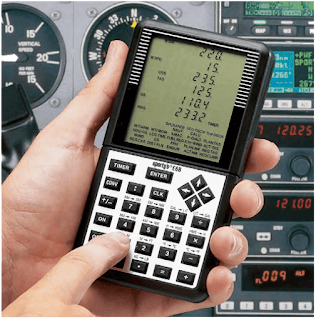Figure 1. Dedicated electronic flight computers are programmed to solve weight and balance problems, as well as flight problems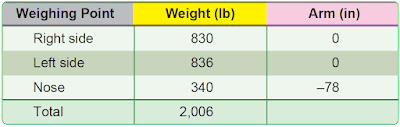Figure 2. Weight and balance data of a typical nosewheel airplane

The problem just solved with an electronic calculator and an E6-B can also be solved with a dedicated flight computer using the information shown in Figure 2. Each flight computer handles the problems in a slightly different way, but all are programmed with prompts that solicit the required data to be inputted so there is no need to memorize any formulas. Weight and arms are inputted as called for, and a running total of the weight, moment, and CG are displayed.

## Typical Weight and Balance Problems

A hand-held electronic calculator like the one in Figure 1 is a valuable tool for solving weight and balance problems. It can be used for a variety of problems and has a high degree of accuracy. The examples given here are solved with a calculator using only the (×),(÷),(+),( – ), and (+/–) functions. If other functions are available on your calculator, some of the steps may be simplified

## Determining CG in Inches From the Datum

This type of problem is solved by firs determining the location of the CG in inches from the main wheel weighing points, then measuring this location in inches from the datum. There are four types of problems involving the location of the CG relative to the datum.

### Nosewheel Airplane With Datum Ahead of the Main Wheels

The datum (D) is 128 inches ahead of the main wheel weighing points; the weight of the nosewheel (F) is 340 pounds, and the distance between main wheels and nosewheel (L) is 78 inches. The total weight (W) of the airplane is 2,006 pounds. Refer to Figure 3.Figure 3. Locating the CG of an airplane relative to the datum

Use this formula:

1. Determine the CG in inches from the main wheel:
(340)(×)(78)(÷)(2006)(=) 13.2

2. Determine the CG in inches form the datum:
(128)(–)(13.2)(=) 114.8
The CG is 114.8 inches behind the datum.

### Nosewheel Airplane With Datum Behind the Main Wheels

The datum (D) is 75 inches behind the main wheel weighing points, the weight of the nosewheel (F) is 340 pounds, and the distance between main wheels and nosewheel (L) is 78 inches. The total weight (W) of the airplane is 2,006 pounds. Refer to Figure 4.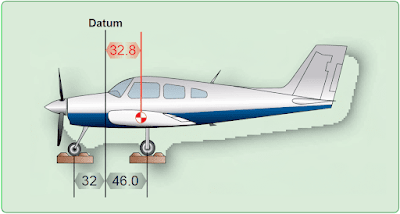Figure 4. The datum is located at the firewall

Use this formula:

1. Determine the CG in inches from the main wheels:
(340)(×)(78)(÷)(2006)(=) 13.2

2. Determine the CG in inches from the datum:
(75)(+)(13.2)(=) 88.2
The minus sign before the parenthesis in the formula means the answer is negative. The CG is 88.2 inches ahead of the datum (–88.2).

### Tailwheel Airplane With Datum Ahead of the Main Wheels

The datum (D) is 7.5 inches ahead of the main wheel weighing points, the weight of the tailwheel (R) is 67 pounds, and the distance between main wheels and tailwheel (L) is 222 inches. The total weight (W) of the airplane is 1,218 pounds. Refer to Figure 5.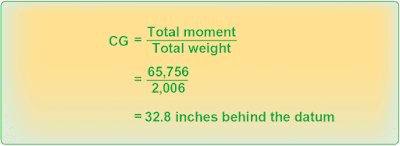Figure 5. Determining the CG

Use this formula:

1. Determine the CG in inches from the main wheels.
(67)(×)(222)(÷)(1218)(=) 12.2

2. Determine the CG in inches from the datum:
(7.5)(+)(12.2)(=) 19.7
The CG is 19.7 inches behind the datum.

### Tailwheel Airplane With Datum Behind the Main Wheels

The datum (D) is 80 inches behind the main wheel weighing points, the weight of the tailwheel (R) is 67 pounds, and the distance between main wheels and tailwheel (L) is 222 inches. The total weight (W) of the airplane is 1,218 pounds. Refer to Figure 6.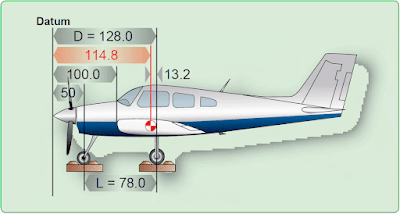Figure 6. The datum is 100 inches forward of the wing root leading edge

Use this formula:

1.Determine the CG in inches from the main wheels:
(67)(×)(222)(÷)(1218)(=) 12.2
2.Determine the CG in inches from the datum:
(80)(+/–)(+)(12.2)(=) –67.8
The CG is 67.8 inches ahead of the datum.

### Determining CG, Given Weights, and Arms

Some weight and balance problems involve weights and arms to determine the moments. Divide the total moment by the total weight to determine the CG. Figure 7 contains the specifications for determining the CG using weights and arms.Figure 7. Specifications for determining the CG of an airplane using weight and arm

Determine the CG by using the data in Figure 7 and following these steps:

1. Determine the total weight and record this number:
(830)(+)(836)(+)(340)(=) 2,006

2. Determine the moment of each weighing point and record them:
(830)(×)(128)(=) 106,240
(836)(×)(128)(=) 107,008
(340)(×)(50)(=) 17,000

3. Determine the total moment and divide this by the total weight:
(106240)(+)(107008)(+)(17000)(=)(÷)(2006)(=) 114.8

This airplane weighs 2,006 pounds and its CG is 114.8 inches from the datum.

## Determining CG, Given Weights, and Moment Indexes

Other weight and balance problems involve weights and moment indexes, such as moment/100 or moment/1,000. To determine the CG, add all the weights and all the moment indexes. Then, divide the total moment index by the total weight and multiply the answer by the reduction factor. Figure 8 contains the specifications for determining the CG using weights and moments indexes.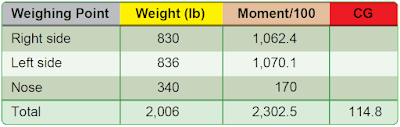Figure 8. Specifications for determining the CG of an airplane using weights and moment indexes

Determine the CG by using the data in Figure 8 and following these steps:

1. Determine the total weight and record this number:
(830)(+)(836)(+)(340)(=) 2,006

2. Determine the total moment index, divide this by the total weight, and multiply it by the reduction factor of 100:
(1062.4)(+)(1070.1)(+)(170)(=)(2302.5)(÷)(2006)(=)(1.148)(×)(100)(=) 114.8

This airplane weighs 2,006 pounds and its CG is 114.8 inches from the datum.

## Determining CG in Percent Mean Aerodynamic Chord (MAC)

• The loaded CG is 42.47 inches aft of the datum.
• MAC is 61.6 inches long.
• LEMAC is at station 20.1.

1. Determine the distance between the CG and LEMAC:
(42.47)(–)(20.1)(=) 22.37

2. Then, use this formula:
(22.37)(×)(100)(÷)(61.6)(=) 36.3

The CG of this airplane is located at 36.3 percent MAC.

## Determining Lateral CG of a Helicopter

For a helicopter, it is often necessary to determine not only the longitudinal CG, but the lateral CG as well. Lateral CG is measured from butt line zero (BL 0). All items and moments to the left of BL 0 are negative, and all those to the right of BL 0 are positive. Figure 9 contains the specifications for determining the lateral CG of a typical helicopter.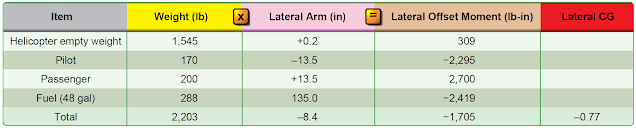Figure 9. Specifications for determining the lateral CG of a helicopter

Determine the lateral CG by using the data in Figure 9 and following these steps:

1. Add all of the weights:
(1545)(+)(170)(+)(200)(+)(288)(=) 2,203

2. Multiply the lateral arm (the distance between butt line zero and the CG of each item) by its weight to get the lateral offset moment of each item. Moments to the right of BL 0 are positive and those to the left are negative.
(1,545)(×)(.2)(=) 309
(170)(×)(13.5)(+/–)(=) –2,295
(200)(×)(13.5)(=) 2,700
(288)(×)(8.4)(+/–)(=) –2,419

3. Determine the algebraic sum of the lateral offset moments.
(309)(+)(2295)(+/–)(+)(2700)(+)(2419)(+/–)(=) –1,705

4. Divide the sum of the moments by the total weight to determine the lateral CG.
(1705)(+/–)(÷)(2203)(=) –0.77

The lateral CG is 0.77 inch to the left of BL0.

## Determining ΔCG Caused by Shifting Weights

Fifty pounds of baggage is shifted from the aft baggage compartment at station 246 to the forward compartment at station 118. The total airplane weight is 4,709 pounds. How much does the CG shift?

1. Determine the number of inches the baggage is shifted:
(246)(–)(118)(=) 128

2. Use this formula:
The CG is shifted forward 1.36 inches.

## Determining Weight Shifted to Cause Specified ΔCG

How much weight must be shifted from the aft baggage compartment at station 246 to the forward compartment at station 118 to move the CG forward 2 inches? The total weight of the airplane is 4,709 pounds.

1. Determine the number of inches the baggage is shifted:

2. Use this formula:
(2)(×)(4709)(÷)(128)(=) 73.6

Moving 73.6 pounds of baggage from the aft compartment to forward compartment shifts the CG forward 2 inches.

## Determining Distance Weight Is Shifted to Move CG a Specific Distance

How many inches aft does a 56 pound battery need to be moved to shift the CG aft by 1.5 inches? The total weight of the airplane is 4,026 pounds.

Use this formula:

## Determining Total Weight of an Aircraft With a Specified ΔCG When Cargo Is Move

What is the total weight of an airplane if moving 500 pounds of cargo 96 inches forward shifts the CG 2.0 inches?

Use this formula:

## Determining Amount of Ballast Needed to Move CG to a Desired Location

How much ballast must be mounted at station 228 to move the CG to its forward limit of +33? The airplane weighs 1,876 pounds and the CG is at +32.2, a distance of 0.8 inch out of limit.

Use this formula: